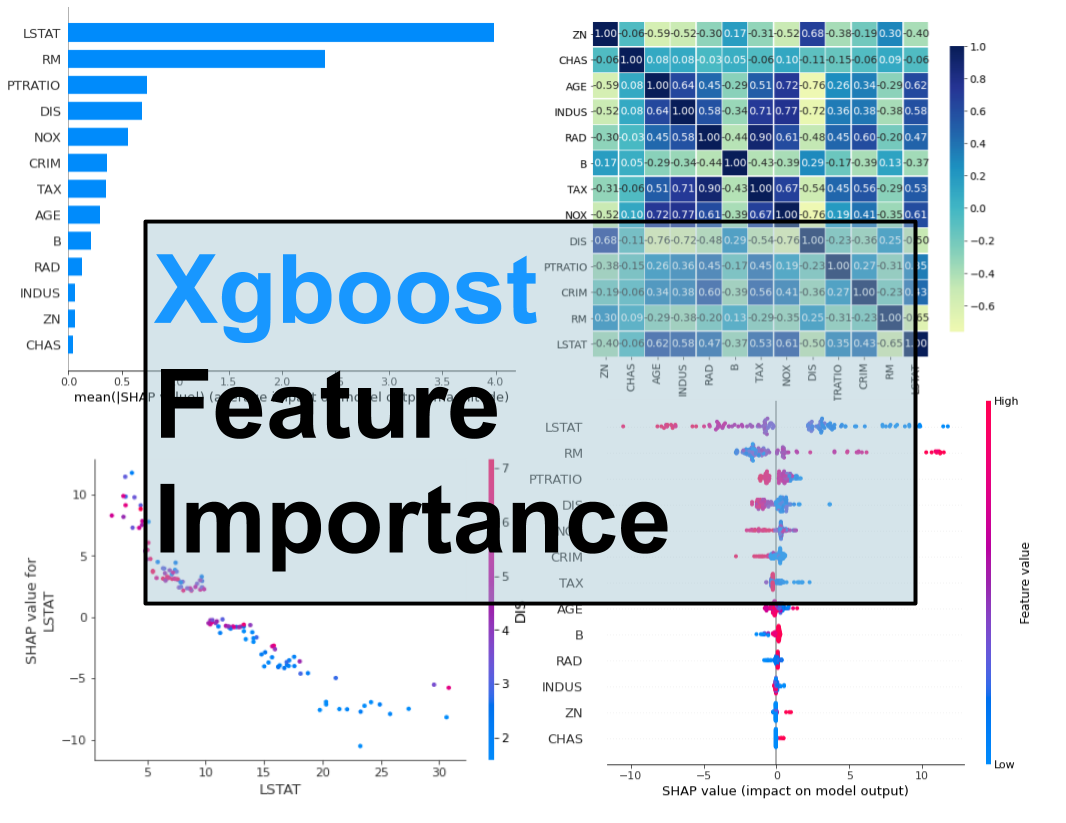• xgboost特征重要性
千次阅读
2019-02-16 21:56:58

from sklearn.model_selection import train_test_split
from sklearn import metrics
from sklearn.datasets  import  make_hastie_10_2
from xgboost.sklearn import XGBClassifier
from xgboost import plot_importance
from matplotlib import pyplot

X, y = make_hastie_10_2(random_state=0)

X_train, X_test, y_train, y_test = train_test_split(X, y, test_size=0.5, random_state=0)##test_size测试集合所占比例

clf = XGBClassifier(
silent=0 ,#设置成1则没有运行信息输出，最好是设置为0.是否在运行升级时打印消息。
learning_rate= 0.3, # 如同学习率
min_child_weight=1,
max_depth=6, # 构建树的深度，越大越容易过拟合
gamma=0,  # 树的叶子节点上作进一步分区所需的最小损失减少,越大越保守，一般0.1、0.2这样子。
subsample=1, # 随机采样训练样本 训练实例的子采样比
max_delta_step=0,#最大增量步长，我们允许每个树的权重估计。
colsample_bytree=1, # 生成树时进行的列采样
reg_lambda=1,  # 控制模型复杂度的权重值的L2正则化项参数，参数越大，模型越不容易过拟合。
n_estimators=100, #树的个数
seed=1000 #随机种子
)

model = clf.fit(X_train,y_train,eval_metric='auc')

y_true, y_pred = y_test, clf.predict(X_test)

print("Accuracy : %.4g" % metrics.accuracy_score(y_true, y_pred))
model.fit(X, y)
print(plot_importance(model))
print(pyplot.show())

更多相关内容
• 面试机器学习相关岗位的时候，**Xgboost** 算法是一个必问项，其中关于特征重要性的问题又是重中之重，一般来说大家仅仅知道两种特征重要性的计算方式，如果你能说出来第三种的话，面试官一定会对你刮目相看。...

面试机器学习相关岗位的时候，Xgboost 算法是一个必问项，其中关于特征重要性的问题又是重中之重，一般来说大家仅仅知道两种特征重要性的计算方式，如果你能说出来第三种的话，面试官一定会对你刮目相看。

本次带你复习和掌握在 Python 中从 Xgboost 模型中获取特征重要性的 3 种方式以备日后面试使用。

• 内置特征重要性
• 基于排列的重要性
• 使用 SHAP 值计算的重要性# Xgboost 内置功能的重要性

import numpy as np
import pandas as pd
import shap

from sklearn.datasets import load_boston

展开全文python 机器学习 数据挖掘 xgboost
• XGBoost中提供了三种特征重要性的计算方法：‘weight’ - the number of times a feature is used to split the data across all trees.‘gain’ - the average gain of the feature when it is used in trees...

在XGBoost中提供了三种特征重要性的计算方法：

‘weight’ - the number of times a feature is used to split the data across all trees.

‘gain’ - the average gain of the feature when it is used in trees

‘cover’ - the average coverage of the feature when it is used in trees

简单来说

weight就是在所有树中特征用来分割的节点个数总和；

gain就是特征用于分割的平均增益

cover 的解释有点晦涩，在[R-package/man/xgb.plot.tree.Rd]有比较详尽的解释：(https://github.com/dmlc/xgboost/blob/f5659e17d5200bd7471a2e735177a81cb8d3012b/R-package/man/xgb.plot.tree.Rd)：the sum of second order gradient of training data classified to the leaf, if it is square loss, this simply corresponds to the number of instances in that branch. Deeper in the tree a node is, lower this metric will be。实际上coverage可以理解为被分到该节点的样本的二阶导数之和，而特征度量的标准就是平均的coverage值。

还是举李航书上那个例子，我们用不同颜色来表示不同的特征，绘制下图展开全文• 1 feature importancegradient boosting 有一个优点是可以...首先要进入两个类：from xgboost import plot_importancefrom matplotlib import pyplot然后在model fit后面添加打印或绘制特征重要性：model.fit(X, y)...

1 feature importance

首先要进入两个类：

from xgboost import plot_importance

from matplotlib import pyplot

然后在model fit后面添加打印或绘制特征重要性：

model.fit(X, y)

plot_importance(model)

pyplot.show()

2 参数优化

XGBoost中提供GridSearchCV方法来对一些重要的超参进行调整，例如：

learning_rate

tree_depth

subsample

n_estimators

max_depth

当然，大多时候学习率的大小一定程度上很影响模型的稳定性，学习率较小容易过拟合，学习率较大又容易欠拟合，所以很多时候都会先考虑对学习率进行调整。

引入如下两个类：

from sklearn.model_selection import GridSearchCV

from sklearn.model_selection import StratifiedKFold

将要尝试的学习率的值放在一个list中即可：

learning_rate = [0.005, 0.001, 0.01, 0.1, 0.2, 0.3]

model = XGBClassifier()

learning_rate = [0.005, 0.001, 0.01, 0.1, 0.2, 0.3]

param_grid = dict(learning_rate=learning_rate)

kfold = StratifiedKFold(n_splits=10, shuffle=True, random_state=7)

grid_search = GridSearchCV(model, param_grid, scoring="neg_log_loss", n_jobs=-1, cv=kfold)

grid_result = grid_search.fit(X, Y)

展开全文• xgboost很好用，但是很多同学对它特征重要性评估的原理不太明白。今天简单梳理下： 特征重要性函数 调用xgb.feature_importances_函数，即可求出各个特种的重要性，这是我们的目标。 现在就来探究一下，这个feature_...
• 特征重要性指标评估三种常用的方式： ①gain 增益意味着相应的特征对通过对模型中的每个树采取每个特征的贡献而计算出的模型的相对贡献。与其他特征相比，此度量值的较高值意味着它对于生成预测更为重要。 ②cover...
• xgboost中的plot_importance方法内置了几种计算重要性的方式。 def plot_importance(booster, ax=None, height=0.2, xlim=None, ylim=None, title='Feature importance', xlabel='F score', ylabel='Features',xgboost weight
• 虚拟性是京剧艺术的重要特征之一阅读附答案-xgboost 特征重要性.docx
• Python中的xgboost可以通过get_fscore获取特征重要性，先看看官方对于这个方法的说明: get_score(fmap=’’, importance_type=‘weight’) Get feature importance of each feature. Importance type can be ...
• XGBoost输出特征重要性以及筛选特征 1，梯度提升算法是如何计算特征重要性的？ 使用梯度提升算法的好处是在提升树被创建后，可以相对直接地得到每个属性的重要性得分。一般来说，重要性分数，衡量了特征在模型中...人工智能 机器学习 python
• xgb_trained_model = xgb.train(params, train_xgb_split, 500, watch_list, early_stopping_rounds=50, verbose_eval=10) importance = xgb_trained_model.get_fscore() temp1 = [] temp2 = [] ...
• 【翻译自 ： Feature Importance and Feature Selection With ... 使用诸如梯度增强之类的决策树方法的集成的好处是，它们可以从训练有素的预测模型中自动提供特征重要性的估计。 在本文中，您将发现如何使用P...
• 使用 XGBoost 特征重要性评分的特征选择 特征重要性分数可用于 scikit-learn 中的特征选择。 这是使用SelectFromModel类完成的，该类采用模型并将数据集转换为具有选定特征的子集。 此类可以采用预先训练的模型，...python 机器学习 xgboost
• xgboost模型对特征重要性进行排序 在这篇文章中，你将会学习到： xgboost对预测模型特征重要性排序的原理（即为什么xgboost可以对预测模型特征重要性进行排序）。 如何绘制xgboost模型得到的特征重要性条形图。...xgboost
• XGBoost特征重要性通过特征在所有弱分类器中节点出现次数来衡量，直接上源码。 该源码读取训练数据采用csv格式，保存特征重要性使用json格式。 一、源码 import json import pandas as pd import xgboost as xgb ...python 机器学习
• 1.xgboost特征重要性排序的原理 xgboost根据结构分数的增益情况计算出来选择哪个特征作为分割点,而某个特征重要性就是它在所有树中出现的次数之和。也就是说一个属性越多的被用来在模型中构建决策树，它的重要性...
• 我们都知道在常见的逻辑回归模型中，每个特征对应一个模型参数wiw_{i}wi​，该参数约大，那么该特征对模型预测结果的影响就会越大，我们就说该特征就越重要，因此LR模型的特征重要性评估方式就是wiw_{i}wi​的大小，...
• 使用诸如梯度增强之类的决策树方法的集成的好处是，它们可以从训练有素的预测模型中自动提供特征重要性的估计。在本文中，您将发现如何使用Python中的XGBoost库来估计特征对于预测性建模问...python 机器学习 人工智能
• 1.输出XGBoost特征重要性from matplotlib import pyplotpyplot.bar(range(len(model_XGB.feature_importances_)), model_XGB.feature_importances_)pyplot.show()from matplotlib import pyplotpyplot.bar(range...
• 使用像梯度增强这样的决策树方法的集合的一个好处是，它们可以从经过训练的预测模型中自动提供特征重要性的估计。 在这篇文章中，您将发现如何使用Python中的XGBoost库估计特性对于预测建模问题的重要性。 读完这篇...python xgboost
•python
• 从具有最高分数的模型特征中进行选择class sklearn.feature_selection.SelectFromModel(estimator, *, threshold=None, prefit=False, norm_order=1, max_features=None) 可选参数： （1）estimator: object，用来...python
• 一、xgboost特征重要性进行排序的原理（即为什么xgboost可以对特行的重要性进行排序） 根据结构分数的增益情况，计算选择哪个特征的哪个分割点；某个特征重要性，就是它在所有树中出现的次数之和。 二、如何...
• XGBOOST模型对于一些场景有着不错的表现，切提供了一个很多的特征重要性的计算： 准备： 把一些用到的包安装一下，如xgboost,sklearn等 建议使用国内的镜像源，否则106M会让你等很久， 具体可以参考： pip...机器学习
• 这里写目录标题 wsl 简介与安装 离线安装wsl 下载wsl 使用 powershell ...[libgomp.so.1] pyspark + xgboost 简单实践【分类+特征重要性】 pipeline 构建 基于随机森林的特征重要性排序 xgboost 特征重要性 参考文献xgboost 机器学习...